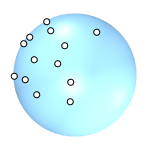# SphereSolid

Sphere >

<Options>

The Sphere command draws a solid sphere.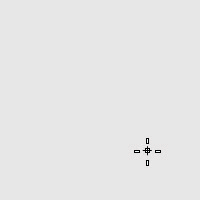Command-line options

Creates a sphere by picking the center point and a radius point.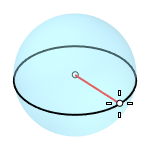### 2Point

Creates a sphere from two opposite points on the base circle.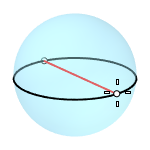### 3Point

Creates a sphere from three points on the base circle.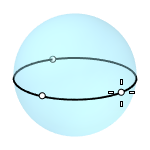### Tangent

Creates a sphere with the base circle tangent to one, two or three curves.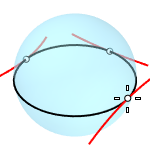### AroundCurve

Creates a sphere from its center point on a curve, and a point on the base circle perpendicular to the curve.### 4Point

Creates a sphere from three points on a section circle and a point on the sphere.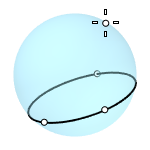### FitPoints

Creates a sphere by fitting to selected point objects, curve and surface control points, and mesh vertices.